# 青南的小世界

::  ::  ::  ::::
 60 随笔 :: 0 文章 :: 136 评论

## 连例子都不举的垃圾文章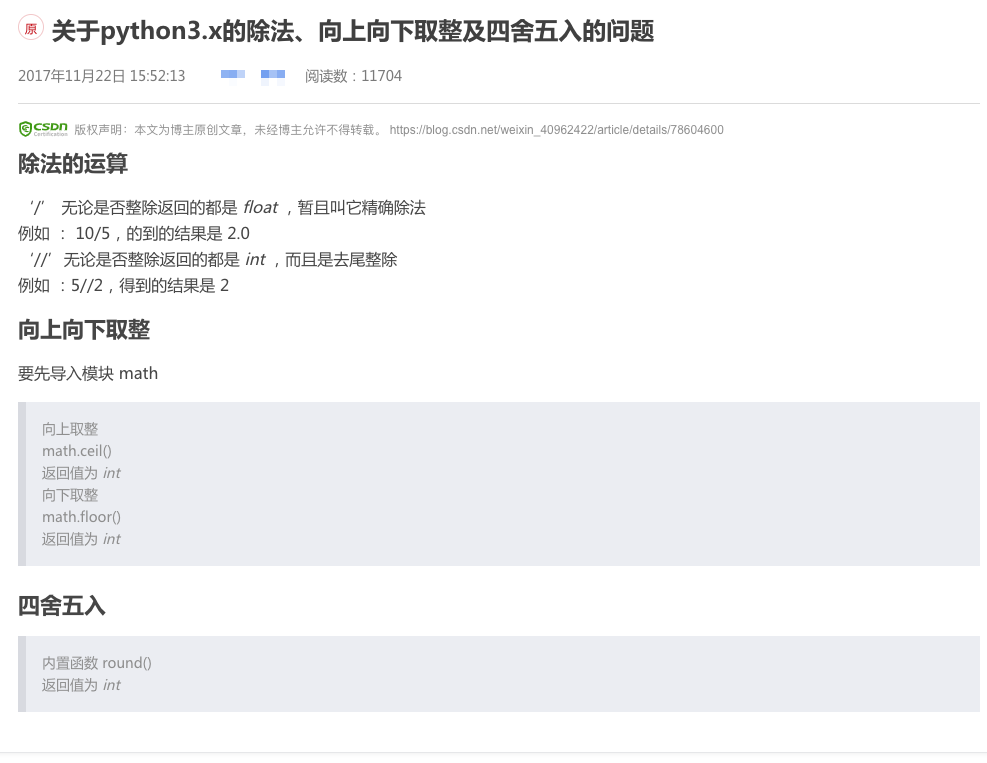## 使用round函数

>>> round(1.234, 2)
1.23


>>> round(11.245, 2)
11.24


## 先放大再缩小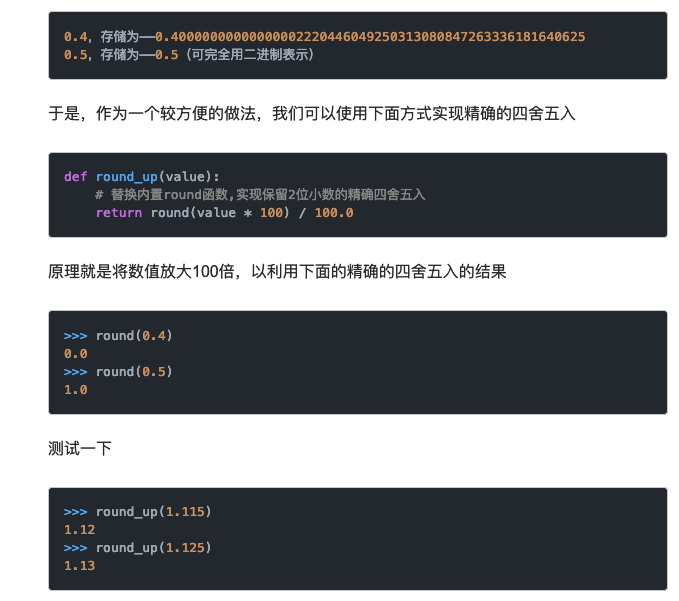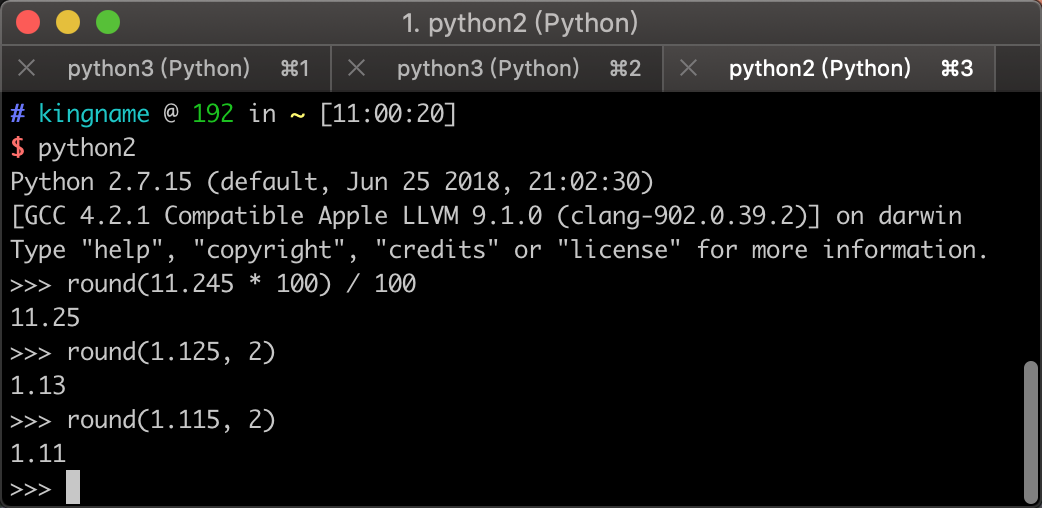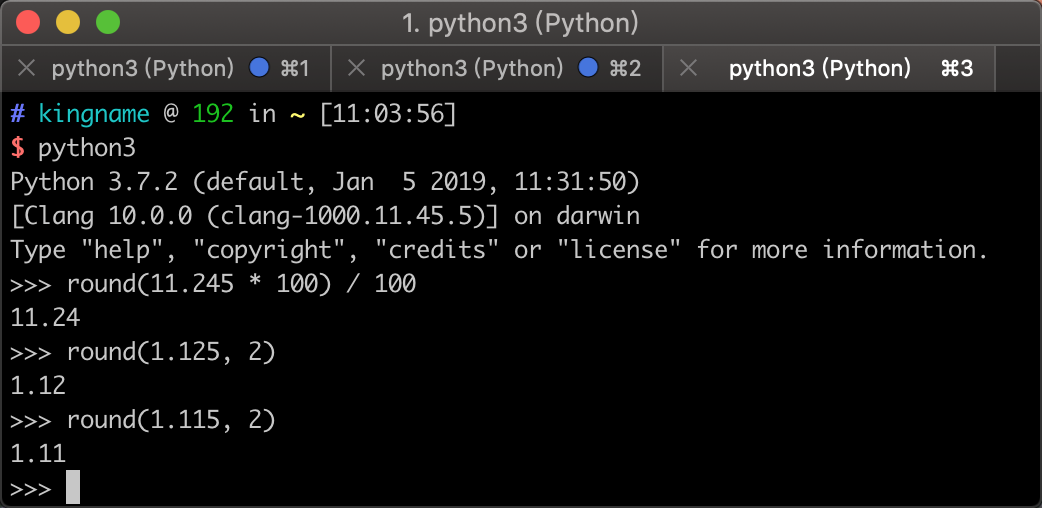### 装逼货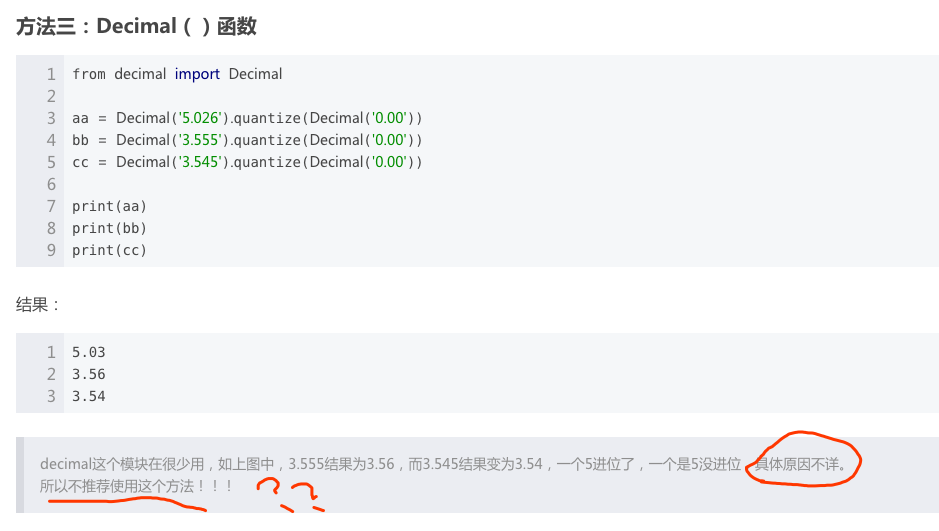具体原因不详 ？？？？

不推荐使用这个方法？？？

decimal是专门为高精度计算用的模块，他竟然说不建议大家使用？？？## round到底出了什么问题？

>>> round(0.125, 2)
0.12


>>> round(0.375, 2)
0.38


1. 如果d小于5，直接舍去
2. 如果d大于5，直接进位
3. 如果d等于5：
1. d后面没有数据，且c为偶数，那么不进位，保留c
2. d后面没有数据，且c为奇数，那么进位，c变成(c + 1)
3. 如果d后面还有非0数字，例如实际上小数为a.bcdef，此时一定要进位，c变成(c + 1)

1. 你的这个小数在计算机中能不能被精确储存？如果不能，那么它可能并没有达到四舍五入的标准，例如1.115，它的小数点后第三位实际上是4，当然会被舍去。
2. 如果你的这个小数在计算机中能被精确表示，那么，round采用的进位机制是奇进偶舍，所以这取决于你要保留的那一位，它是奇数还是偶数，以及它的下一位后面还有没有数据。

## 如何正确进行四舍五入

>>>Decimal('1.41421356').quantize(Decimal('1.000'))
Decimal('1.414')


>>> from decimal import Decimal
>>> Decimal('0.125').quantize(Decimal('0.00'))
Decimal('0.12')
>>> Decimal('0.375').quantize(Decimal('0.00'))
Decimal('0.38')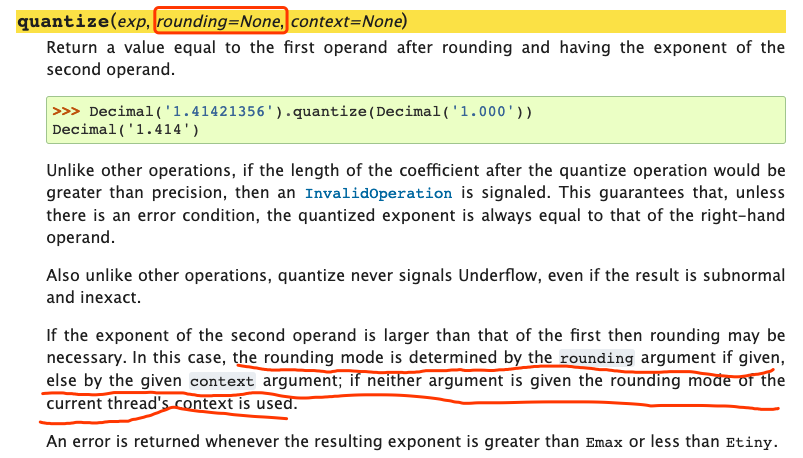>>> from decimal import getcontext
>>> getcontext().rounding
'ROUND_HALF_EVEN'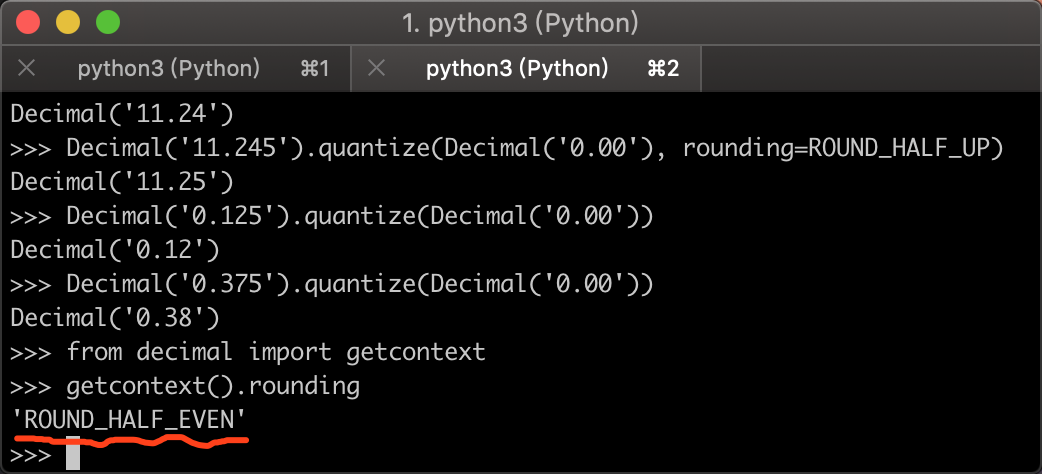ROUND_HALF_EVEN实际上就是奇进偶舍！如果要指定真正的四舍五入，那么我们需要在quantize中指定进位方式为ROUND_HALF_UP

>>> from decimal import Decimal, ROUND_HALF_UP
>>> Decimal('0.375').quantize(Decimal('0.00'), rounding=ROUND_HALF_UP)
Decimal('0.38')
>>> Decimal('0.125').quantize(Decimal('0.00'), rounding=ROUND_HALF_UP)
Decimal('0.13')



>>> Decimal(0.375).quantize(Decimal('0.00'), rounding=ROUND_HALF_UP)
Decimal('0.38')
>>> Decimal(0.125).quantize(Decimal('0.00'), rounding=ROUND_HALF_UP)
Decimal('0.13')


>>> Decimal(11.245).quantize(Decimal('0.00'), rounding=ROUND_HALF_UP)
Decimal('11.24')
>>> Decimal('11.245').quantize(Decimal('0.00'), rounding=ROUND_HALF_UP)
Decimal('11.25')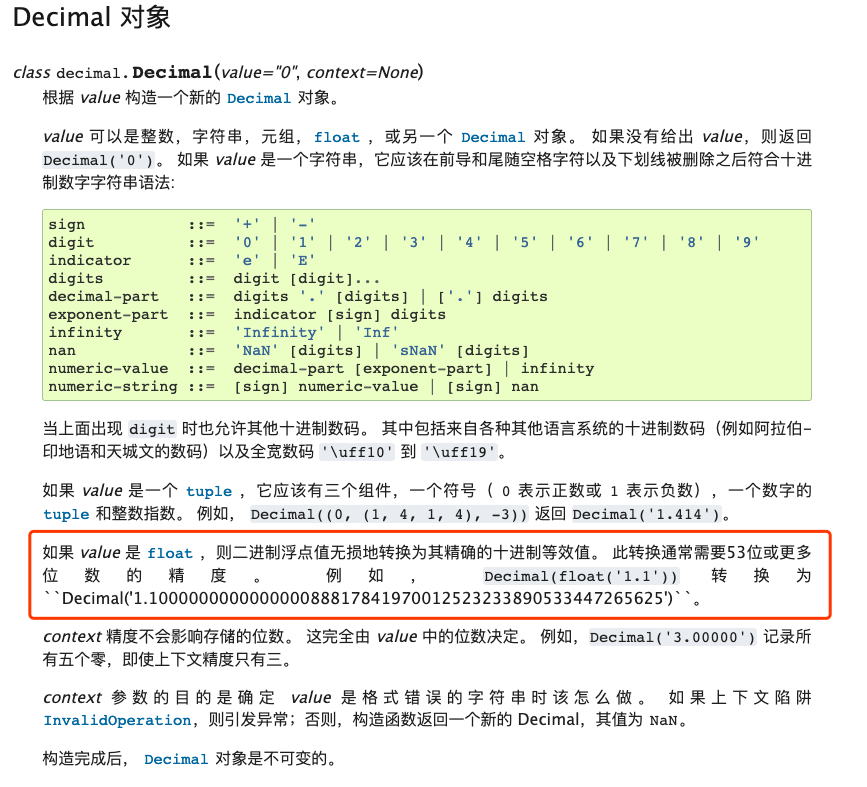from decimal import Decimal, ROUND_HALF_UP

origin_num = Decimal('11.245')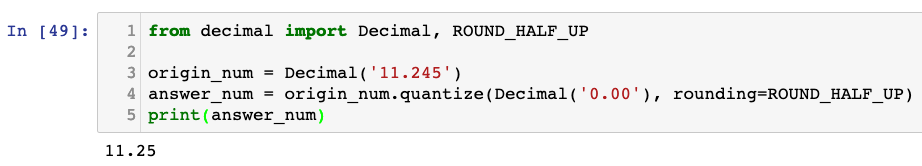a = Decimal('0.1')
b = Decimal('0.2')
c = a + b
print(c)


posted on 2019-03-31 13:13  青南  阅读(2153)  评论(11编辑  收藏